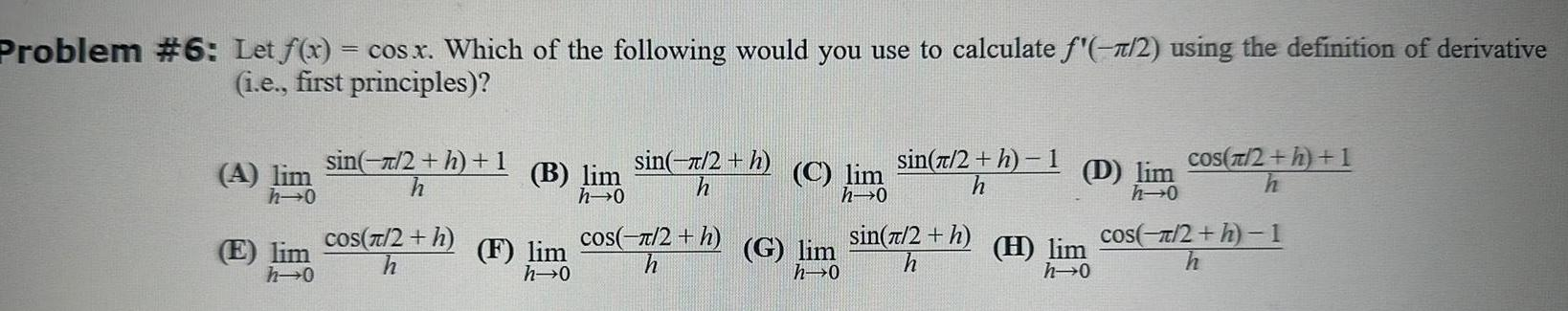Question:

# Problem 6 Let f x cos x Which of the following would you use

Last updated: 9/17/2023Problem 6 Let f x cos x Which of the following would you use to calculate 2 using the definition of derivative i e first principles A E h 0 h 0 sin 2 h 1 h cos 2 h B lim F lim h 0 sin 2 h h os 2 h C lim h 0 G lim h 0 sin 2 h 1 h sin 2 h h D lim h 0 H lim h 0 cos 2 h 1 cos 2 h 1